NEET  >  31 Year NEET Previous Year Questions: Kinetic Theory

# 31 Year NEET Previous Year Questions: Kinetic Theory

Test Description

## 19 Questions MCQ Test Physics Class 11 | 31 Year NEET Previous Year Questions: Kinetic Theory

31 Year NEET Previous Year Questions: Kinetic Theory for NEET 2022 is part of Physics Class 11 preparation. The 31 Year NEET Previous Year Questions: Kinetic Theory questions and answers have been prepared according to the NEET exam syllabus.The 31 Year NEET Previous Year Questions: Kinetic Theory MCQs are made for NEET 2022 Exam. Find important definitions, questions, notes, meanings, examples, exercises, MCQs and online tests for 31 Year NEET Previous Year Questions: Kinetic Theory below.
Solutions of 31 Year NEET Previous Year Questions: Kinetic Theory questions in English are available as part of our Physics Class 11 for NEET & 31 Year NEET Previous Year Questions: Kinetic Theory solutions in Hindi for Physics Class 11 course. Download more important topics, notes, lectures and mock test series for NEET Exam by signing up for free. Attempt 31 Year NEET Previous Year Questions: Kinetic Theory | 19 questions in 40 minutes | Mock test for NEET preparation | Free important questions MCQ to study Physics Class 11 for NEET Exam | Download free PDF with solutions
 1 Crore+ students have signed up on EduRev. Have you?
31 Year NEET Previous Year Questions: Kinetic Theory - Question 1

### Two containers A and B are partly filled with water and closed. The volume of A is twice that of B and it contains half the amount of water in B. If both are at the same temperature, the water vapour in the containers will have pressure in the ratio of 

Detailed Solution for 31 Year NEET Previous Year Questions: Kinetic Theory - Question 1

Vapour pressure  does not depend on the amount of substance. It depends on the temperature alone.

31 Year NEET Previous Year Questions: Kinetic Theory - Question 2

### At constant volume, temperature is increased then

Detailed Solution for 31 Year NEET Previous Year Questions: Kinetic Theory - Question 2

As the temperature increases, the average velocity increases. So, the collisions are faster.

31 Year NEET Previous Year Questions: Kinetic Theory - Question 3

### A polyatomic gas with n degrees of freedom has a mean energy per molecule given by 

Detailed Solution for 31 Year NEET Previous Year Questions: Kinetic Theory - Question 3

According to law of equipartition of energy, the energy per degree of freedom is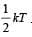For a polyatomic gas with n degrees of freedom, the mean energy per molecule  =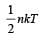31 Year NEET Previous Year Questions: Kinetic Theory - Question 4

For a certain gas the ratio of specific heats is given to be γ = 1.5. For this gas 

Detailed Solution for 31 Year NEET Previous Year Questions: Kinetic Theory - Question 4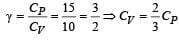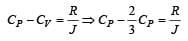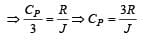31 Year NEET Previous Year Questions: Kinetic Theory - Question 5

According to kinetic theory of gases, at absolute zero temperature 

Detailed Solution for 31 Year NEET Previous Year Questions: Kinetic Theory - Question 5

The average kinetic energy of a gas molecular is directly eruptional to absolute temperature only; this implies that all molecular motion stops if the temperature is reduced to absolute zero.

31 Year NEET Previous Year Questions: Kinetic Theory - Question 6

One mole of an ideal monoatomic gas requires 207 J heat to raise the temperature by 10 K when heated at constant pressure. If the same gas is heated at constant volume to raise the temperature by the same 10 K, the heat required is [Given the gas constant R = 8.3 J/ mol. K] 

Detailed Solution for 31 Year NEET Previous Year Questions: Kinetic Theory - Question 6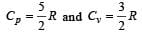We know that  Qv = nCvΔT and Qp = nCpΔT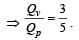Given Qp = 207 J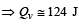31 Year NEET Previous Year Questions: Kinetic Theory - Question 7

Relation between pressure (P) and energy (E) of a gas is 

Detailed Solution for 31 Year NEET Previous Year Questions: Kinetic Theory - Question 7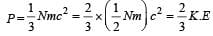31 Year NEET Previous Year Questions: Kinetic Theory - Question 8

Three containers of the same volume contain three different gases. The masses of the molecules are m1, m2 and m3 and the number of molecules in their respective containers are N1, N2 and N3. The gas pressure in the containers are P1, P2 and P3 respectively. All the gases are now mixed and put in one of these containers.The pressure P of the mixture will be 

Detailed Solution for 31 Year NEET Previous Year Questions: Kinetic Theory - Question 8

According to Dalton’s law of partial pressures, we have  P = P1 + P2 + P3

31 Year NEET Previous Year Questions: Kinetic Theory - Question 9

The number of translational degrees of freedom for a diatomic gas is 

Detailed Solution for 31 Year NEET Previous Year Questions: Kinetic Theory - Question 9

Number of translational degrees of freedom are same for all types of gases.

31 Year NEET Previous Year Questions: Kinetic Theory - Question 10

The temperature of a gas is raised from 27°C to 927°C.The root mean square speed is 

Detailed Solution for 31 Year NEET Previous Year Questions: Kinetic Theory - Question 10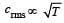As temperature increases from 300 K to 1200 K that is four times, so, crms will be doubled.

31 Year NEET Previous Year Questions: Kinetic Theory - Question 11

The equation of state, corresponding to 8g of O2 is

Detailed Solution for 31 Year NEET Previous Year Questions: Kinetic Theory - Question 11

8g of oxygen is equivalent to (1/4) mole.

31 Year NEET Previous Year Questions: Kinetic Theory - Question 12

If Cs be the velocity of sound in air and C be the r.m.s velocity, then 

Detailed Solution for 31 Year NEET Previous Year Questions: Kinetic Theory - Question 12

Velocity of sound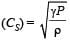R.M.S. velocity of gas molecules =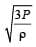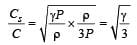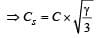31 Year NEET Previous Year Questions: Kinetic Theory - Question 13

At 0 K, which of the following properties of a gas will be zero? 

Detailed Solution for 31 Year NEET Previous Year Questions: Kinetic Theory - Question 13

At 0 K, molecular motion stops. Hence, kinetic energy of molecules becomes zero.

31 Year NEET Previous Year Questions: Kinetic Theory - Question 14

The degree of freedom of a molecule of a triatomic ga s is

Detailed Solution for 31 Year NEET Previous Year Questions: Kinetic Theory - Question 14

No. of degree of freedom = 3 K – N where K is no. of atom and N is the number of relations between atoms. For triatomic gas, K = 3, N = 3C2 = 3
No. of degree of freedom = 3 (3) – 3 = 6

31 Year NEET Previous Year Questions: Kinetic Theory - Question 15

The equation of state for 5 g of oxygen at a pressure P and temperature T, when occupying a volume V, will be 

Detailed Solution for 31 Year NEET Previous Year Questions: Kinetic Theory - Question 15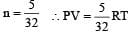[∵ PV = nRT]

31 Year NEET Previous Year Questions: Kinetic Theory - Question 16

At 10° C the value of the density of a fixed mass of an ideal gas divided by its pressure is x. At 110°C this ratio is: 

Detailed Solution for 31 Year NEET Previous Year Questions: Kinetic Theory - Question 16

Let the mass of the gas be m.
At a fixed temperature and pressure, volume is fixed.
Density of the gas,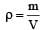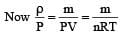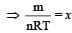(By question)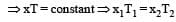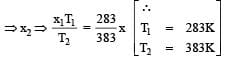31 Year NEET Previous Year Questions: Kinetic Theory - Question 17

In the given (V – T) diagram, what is the relation between pressure P1 and P2 ? [NEET 2013]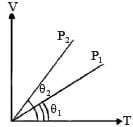Detailed Solution for 31 Year NEET Previous Year Questions: Kinetic Theory - Question 17

P1 > P2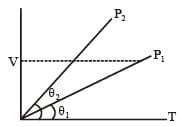As V = constant ⇒ P ∝ T
Hence from V–T graph P1 > P2

31 Year NEET Previous Year Questions: Kinetic Theory - Question 18

The amount of heat energy required to raise the temperature of 1g of Helium at NTP, from T1K to T2K is [NEET 2013]

Detailed Solution for 31 Year NEET Previous Year Questions: Kinetic Theory - Question 18

From first law of thermodynamics ΔQ = ΔU + ΔW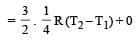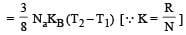31 Year NEET Previous Year Questions: Kinetic Theory - Question 19

In a vessel, the gas is at a pressure P. If the mass of all the molecules is halved and their speed is doubled, then the resultant pressure will be [NEET Kar. 2013]

Detailed Solution for 31 Year NEET Previous Year Questions: Kinetic Theory - Question 19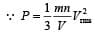When mass is halved and speed is doubled then Resultant pressure,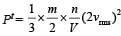= 2 P.

## Physics Class 11

126 videos|449 docs|210 tests
 Use Code STAYHOME200 and get INR 200 additional OFF Use Coupon Code
Information about 31 Year NEET Previous Year Questions: Kinetic Theory Page
In this test you can find the Exam questions for 31 Year NEET Previous Year Questions: Kinetic Theory solved & explained in the simplest way possible. Besides giving Questions and answers for 31 Year NEET Previous Year Questions: Kinetic Theory, EduRev gives you an ample number of Online tests for practice

## Physics Class 11

126 videos|449 docs|210 tests

### How to Prepare for NEET

Read our guide to prepare for NEET which is created by Toppers & the best Teachers